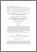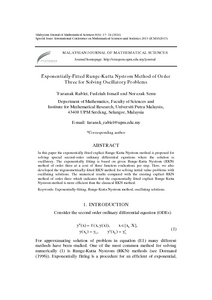# Exponentially-fitted Runge-Kutta Nystrom method of order three for solving oscillatory problems

## Citation

Rabiei, Faranak and Ismail, Fudziah and Senu, Norazak (2014) Exponentially-fitted Runge-Kutta Nystrom method of order three for solving oscillatory problems. Malaysian Journal of Mathematical Sciences, 8 (S). pp. 17-24. ISSN 1823-8343; ESSN: 2289-750X

## Abstract

In this paper the exponentially fitted explicit Runge-Kutta Nystrom method is proposed for solving special second-order ordinary differential equations where the solution is oscillatory. The exponentially fitting is based on given Runge-Kutta Nystrom (RKN) method of order three at a cost of three function evaluations per step. Here, we also developed the trigonometrically-fitted RKN method for solving initial value problems with oscillating solutions. The numerical results compared with the existing explicit RKN method of order three which indicates that the exponentially fitted explicit Runge-Kutta Nystrom method is more efficient than the classical RKN method.Preview
PDF
39047.pdfView Item